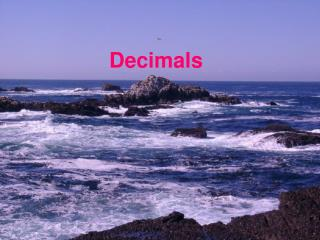DownloadDownload PresentationDecimals

# Decimals

Télécharger la présentation## Decimals

- - - - - - - - - - - - - - - - - - - - - - - - - - - E N D - - - - - - - - - - - - - - - - - - - - - - - - - - -
##### Presentation Transcript

1. Decimals

2. To Do: A restaurant offers a 10% discount. Do you prefer getting the discount before or after the 8% sales tax is added on? First the discount, then the tax: P 0.9  1.08 First the tax, then the discount: P 1.08  0.9 Same

3. Think about how you might respond to a student who asks you the following question? Adding and Multiplying Decimals Why, when we add decimals, we have to line up the decimal points, while, when we multiply decimals, we don’t have to line up the decimal points?

4. To Do: Add 0.3 + 0.4 by first expressing each as a fraction, then adding the fractions, and finally convert back to a decimal. How does this compare with the way decimal addition usually is done?

5. To Do: Add 0.6 + 0.9 by first expressing each as a fraction. How does this compare with the way decimal addition usually is done?

6. To Do: Add 0.3 + 0.57 by first expressing each as a fraction. How does this compare with the way decimal addition usually is done?

7. To Do: Multiply 0.7×0.43 by first expressing each as a fraction. How does this compare with the way decimal multiplication usually is done?

8. Terminating and Repeating Decimals Answer: If the only prime divisors of n are 2 and/or 5.

9. Answer: If there is a prime divisor of n other than 2 and 5.

10. When 10, 20, 30, 40, 50 or 60 is divided by 7, the remainder can only be one of the numbers 1, 2, 3, 4, 5 or 6, which means we’ll again be dividing into either 10, 20, 30, 40, 50 or 60.

11. To Do: What is the smallest number of the form 9999 for which 7 is a divisor?

12. To Do: What is the smallest number of the form 9999 for which 13 is a divisor? To Do: What is the smallest number of the form 9999 for which 37 is a divisor?

13. 99999 = 32 41  271

14. Why does this rule work?

15. Thus, their decimal parts must be equal. That is, g = a, h = b, i = c, j = d, k = e, and l = f. Therefore, the decimal repeats every 6 decimal places.